Adaptive lasso selection is a modification of lasso selection; in adaptive lasso selection weights are applied to each of the parameters in forming the lasso constraint (Zou, 2006). More precisely, suppose that the response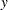has mean zero and the regressorsare scaled to have mean zero and common standard deviation. Furthermore, suppose that you can find a suitable estimator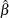of the parameters in the true model and you define a weight vector by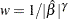, where
. Then the adaptive lasso regression coefficients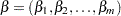are the solution to the constrained optimization problem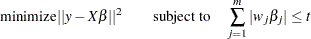You can specifyby using the INEST=suboption of the SELECTION=LASSO option in the MODEL statement. The INEST= data set has the same structure as the OUTEST= data set that is produced by several SAS/STAT procedures including the REG and LOGISTIC procedures. The INEST= data set must contain all explanatory variables in the MODEL statement. It must also contain an intercept variable named Intercept unless the NOINT option is specified in the MODEL statement. If BY processing is used, the INEST= data set must also include the BY variables, and there must be one observation for each BY group. If the INEST= data set also contains the _TYPE_ variable, only observations with _TYPE_ value 'PARMS' are used.
If you do not specify an INEST= data set, then PROC GLMSELECT uses the solution to the unconstrained least squares problem as the estimator. This is appropriate unless collinearity is a concern. If the regressors are collinear or nearly collinear, then Zou (2006) suggests using a ridge regression estimate to form the adaptive weights.